In :
%matplotlib inline
from collections import namedtuple
import itertools
import matplotlib.pyplot as plt
import seaborn as sns
import numpy as np
import datetime as dt
import scipy.stats as stats
from scipy.interpolate import interp1d
import scipy
import statsmodels.api as sm

from sumrv import SumRv

bigfontsize=20
labelfontsize=16
tickfontsize=16
sns.set_context('talk')
plt.rcParams.update({'font.size': bigfontsize,
'axes.labelsize':labelfontsize,
'xtick.labelsize':tickfontsize,
'ytick.labelsize':tickfontsize,
'legend.fontsize':tickfontsize,
})


# Computing the PDF of a summed 1D random variate in Python¶

Suppose we want to model a random variate $Y$, such that $$Y = X + E$$ where $X$ is some random variate of interest, and $E$ represents an additive error term.

Of course, we can model this using basic Monte-Carlo methods - run many samplings over both $X$ and $E$, summing the results (this has the advantage of being trivially generalisable to N dimensions). But if we're interested in the precise shape of $Y's$ PDF, this can be terribly inefficient. For example, if $X$ is not a standard random variate but instead a computationally intensive transform of one, then we might want to minimise the number of samplings of $X$ (I'm assuming the transform has a well behaved parametric form).

We can determine the PDF of $Y$ analytically via the convolution of the component variate PDFs: $$P(Y) = P(X)*P(E)$$

It's worth noting that there are a bunch of standard analytical solutions, but often these don't apply.

## Convolution using Scipy ¶

We can approximately compute the general case using Scipy's convolution and interpolation routines. First, a basic implementation to give an idea of what's going on:

In :
simple = stats.uniform(loc=2,scale=3)
errscale = 0.25
err = stats.norm(loc=0,scale=errscale)

# NB Kernel support array **MUST** be symmetric about centre of the kernel (error PDF) for this to work right.
# Support also needs to extend about any significant areas of the component PDFs.
# Here, we just define one massive support for both input PDF, and error PDF (kernel)
# But we can do much better (see later)

#NB step-size determines precision of approximation
delta = 1e-4
big_grid = np.arange(-10,10,delta)

# Cannot analytically convolve continuous PDFs, in general.
# So we now make a probability mass function on a fine grid
# - a discrete approximation to the PDF, amenable to FFT...
pmf1 = simple.pdf(big_grid)*delta
pmf2 = err.pdf(big_grid)*delta
conv_pmf = scipy.signal.fftconvolve(pmf1,pmf2,'same') # Convolved probability mass function
print "Grid length, sum(gauss_pmf), sum(uni_pmf),sum(conv_pmf):"
print len(big_grid), sum(err.pdf(big_grid)*delta), sum(simple.pdf(big_grid)*delta), sum(conv_pmf)
conv_pmf = conv_pmf/sum(conv_pmf)

plt.plot(big_grid,pmf1, label='Tophat')
plt.plot(big_grid,pmf2, label='Gaussian error')
plt.plot(big_grid,conv_pmf, label='Sum')
plt.xlim(-3,max(big_grid))
plt.legend(loc='best'), plt.suptitle('PMFs')

Grid length, sum(gauss_pmf), sum(uni_pmf),sum(conv_pmf):
200000 1.0 1.0 1.0

Out:
(<matplotlib.legend.Legend at 0x8223790>, <matplotlib.text.Text at 0x8212710>)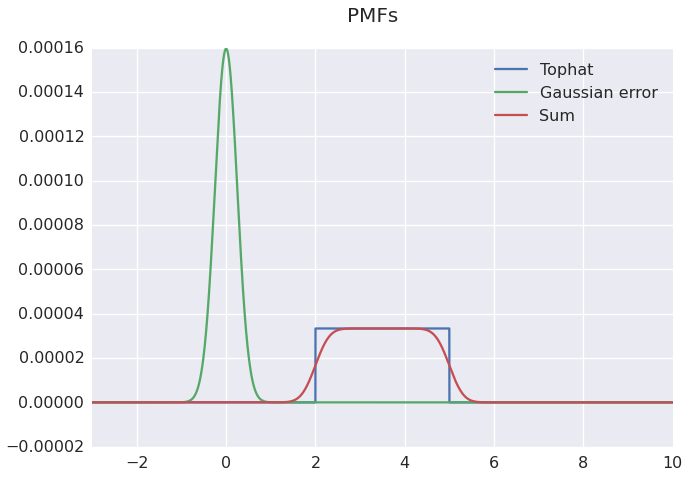All that remains is to convert back from the resulting PMF to a PDF: renormalise (divide by delta), and then interpolate to provide a continuous approximation.

Incidentally, you definitely want to use fftconvolve, the regular version is $\sim100$ times slower:

In :
print "FFT timings:"
%timeit conv_pmf = scipy.signal.fftconvolve(pmf1,pmf2,'same') # Convolved probability mass function
#print "Non-FFT timings:" ## Will take a while...
#%timeit slow_conv_pmf = scipy.signal.convolve(pmf1,pmf2,'same') # Convolved probability mass function

FFT timings:
10 loops, best of 3: 37.3 ms per loop


I've put together a slightly slicker version of the above code as a class inheriting from scipy.stats.rv_continuous, but the principle is the same. I've uploaded the source along with this notebook. Here it is in action:

In :
sum_rv_delta_size = 1e-2
sum_rv = SumRv(simple, err, delta=sum_rv_delta_size, dist_truncation=0.0)
new_grid = np.linspace(-2,7,2**10)
plt.plot(new_grid,simple.pdf(new_grid), label='Tophat')
plt.plot(new_grid,err.pdf(new_grid), label='Gaussian error')
plt.plot(new_grid,sum_rv.pdf(new_grid), label='Sum')
#plt.xlim(-3,8)
plt.legend(loc='best'), plt.suptitle('Discretization and convolution via scipy routines')

Out:
(<matplotlib.legend.Legend at 0x7f57b50>, <matplotlib.text.Text at 0x7f46450>)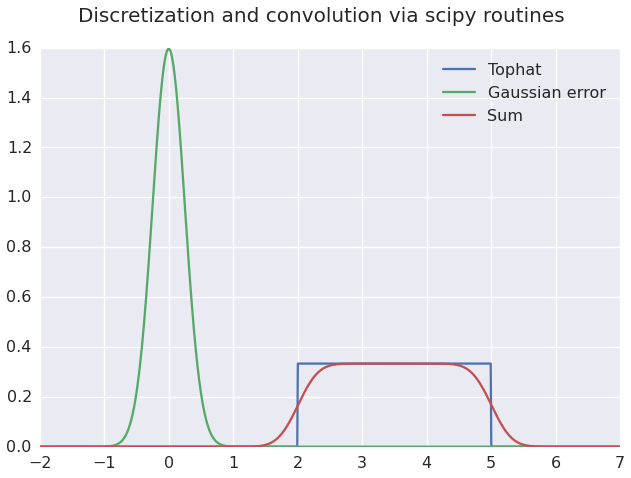The class also takes care of computing the CDF correctly:

In :
plt.plot(new_grid, simple.cdf(new_grid), label='Tophat')
plt.plot(new_grid, err.cdf(new_grid), label='Gaussian error')
plt.plot(new_grid, sum_rv.cdf(new_grid), label='Sum')
plt.xlim(min(new_grid),max(new_grid))
plt.legend(loc='best'), plt.suptitle('CDFs')
plt.ylim(-0.1,1.1)

Out:
(-0.1, 1.1)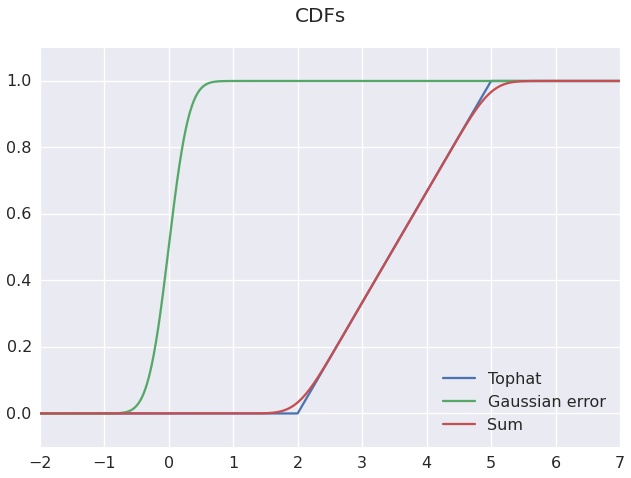## Convolution using Statsmodels KDEs¶

If our error variate $E$ has a distribution in the list of statsmodels Univariate KDE kernels, i.e.:

        - "biw" for biweight
- "cos" for cosine
- "epa" for Epanechnikov
- "gau" for Gaussian.
- "tri" for triangular
- "triw" for triweight
- "uni" for uniform



Then we can use the ready-made KDE functionality from statsmodels, which is more robust, but slower, since it does not rely upon regularly spaced samples of $X$. First, a sanity check on the KDE parameters:

In :
# Demonstrate use of KDEUnivariate to plot kernel shape,
# by running on single sample point:
sample=np.zeros(1)
print "A single sample for our KDE:", sample
kernel = sm.nonparametric.KDEUnivariate(sample)
sigma=2.5
kernel.fit(bw=sigma) # kernel='gau' by default
grid = np.linspace(-5*sigma,5*sigma,2**10)
plt.plot(grid, kernel.evaluate(grid), label='KDE')

#Plot a scipy.stats Gaussian for comparison:
norm = stats.norm(loc=0,scale=sigma)
plt.plot(grid, norm.pdf(grid), label='Normal')
plt.legend()
plt.gcf().suptitle('Gaussian KDE profile of a single sample')
print "Max diff between KDE fit and Gaussian PDF:",max(kernel.evaluate(grid) - norm.pdf(grid))
#%timeit kernel.evaluate(grid)
#%timeit norm.pdf(grid)

A single sample for our KDE: [ 0.]
Max diff between KDE fit and Gaussian PDF: 2.77555756156e-17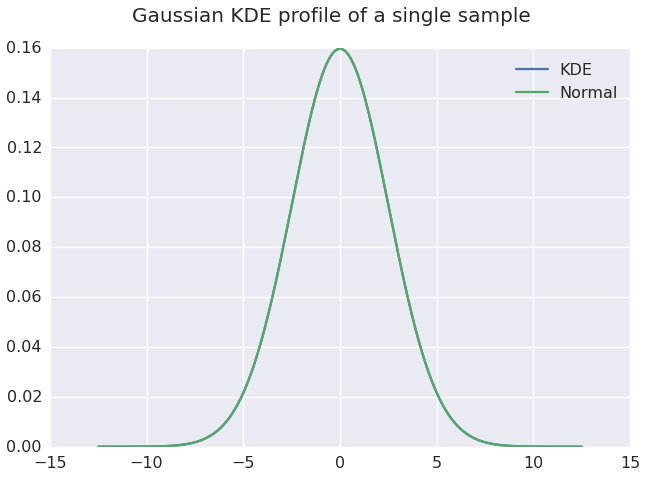## Importance sampling with a weighted KDE¶

Now we've established that the statsmodels KDE kernel behaves as expected, we can employ the weighted-KDE technique to generate a smoothly convolved PDF using importance sampling. I've used roughly the same number of PDF evaluations as for the direct (SciPy) convolution approach, but the requirement that the KDE-fit be weighted means we're restricted to using the inefficient non-FFT implementation. Note that the weighted-KDE fit is broken in statsmodels 0.5.0, but has been fixed in the current dev-version (0.6-dev).

In :
#Generate regular samples of input PDF, weighted by PDF value:
x_dist = stats.uniform(loc=2,scale=3)
w_kde_delta=sum_rv_delta_size*2
sample_locs = np.arange(1,6,w_kde_delta)
print "Weighted KDE sample spacing:", w_kde_delta, ", resulting in", len(sample_locs), "samples."
sample_weights = x_dist.pdf(sample_locs)

weighted_kde = sm.nonparametric.KDEUnivariate(sample_locs)
weighted_kde.fit(weights=sample_weights, bw=errscale,fft=False, kernel='gau')
plt.plot(grid,simple.pdf(grid), label='uniform')
plt.plot(grid,err.pdf(grid), label='err')
plt.plot(weighted_kde.support,weighted_kde.density, label='kde')
# plt.plot(grid,weighted_kde.evaluate(grid), label='kde')
plt.xlim(-2,8)
plt.legend(loc='best'), plt.suptitle('Importance sampling and statsmodels weighted-KDE')

Weighted KDE sample spacing: 0.02 , resulting in 250 samples.

Out:
(<matplotlib.legend.Legend at 0x7431f10>, <matplotlib.text.Text at 0x7438e10>)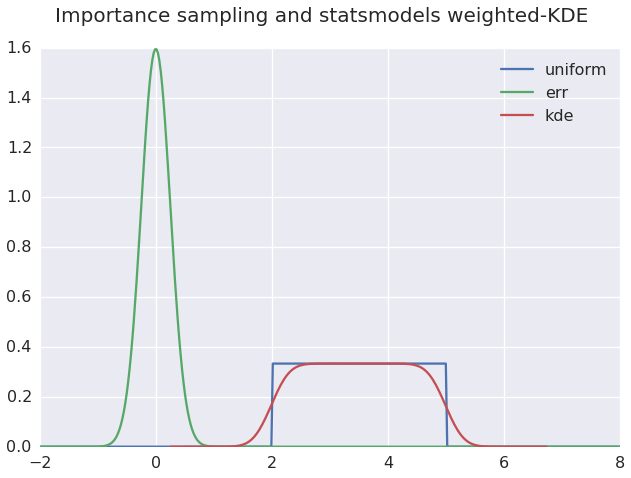In :
# weighted_kde.support


## Monte-Carlo sampling and an FFT-KDE¶

At the risk of getting an unrepresentative sampling of $X$ (and hence a poor-quality approximate convolution), we can try utilising the FFT-KDE approach on a basic Monte-Carlo sample. If our error-variate PDF is narrow, this will be a poor approximation without a large number of samples, but if the error is large then the additional smoothing means we can (perhaps) get away with a relatively small sample size, which effectively means that we don't require detailed knowledge of the PDF of $X$. We can then efficiently sample from this smoothed PDF repeatedly, without requiring further direct samples from $X$. The technique also generalizes trivially to higher dimensions.

In :
x_sample = x_dist.rvs(size=len(sample_locs)*5)
fft_kde = sm.nonparametric.KDEUnivariate(x_sample)
fft_kde.fit(bw=errscale,fft=True)
plt.plot(grid,simple.pdf(grid), label='uniform')
plt.plot(grid,err.pdf(grid), label='err')
plt.plot(grid,fft_kde.evaluate(grid), label='kde')
plt.xlim(-2,8)
plt.legend(loc='best'), plt.suptitle('Basic MC-sampling and statsmodels FFT-KDE')

Out:
(<matplotlib.legend.Legend at 0x8897ed0>, <matplotlib.text.Text at 0x887a190>)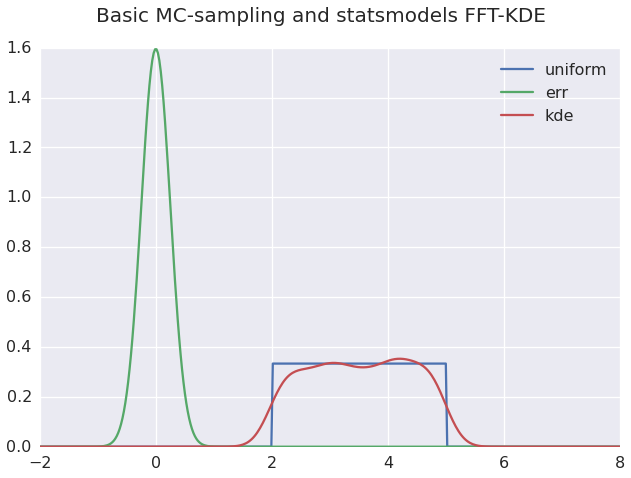## Performance¶

Note there's a big performance difference between these approaches. I've demonstrated the trade-off between initialization and evaluation times below. This comparison is highly unscientific, applying an accuracy criteria of 'does the graph look about the right shape.' In the case of direct Monte-Carlo samples the graph looks wobbly even at 5 times the number of samples used for the weighted KDE, but this would improve somewhat for a wider error distribution.

More to the point, synthetic benchmarks can be misleading - in a real application you will also need to consider the time it takes to sample $X$ or evaluate the PDF of $X$, plus your required level of accuracy. So take the numbers below with a generous pinch of salt - they perhaps give an idea of orders of magnitude, at best.

### Initialization times¶

Scipy convolution (note this includes the evaluation time for the PDF of $X$, but that's probably negligible in comparison to the convolution):

In :
%%timeit
SumRv(simple, err, delta=sum_rv_delta_size)

1000 loops, best of 3: 1.23 ms per loop


Weighted KDE

In :
%%timeit
weighted_kde = sm.nonparametric.KDEUnivariate(sample_locs)
weighted_kde.fit(weights=sample_weights, bw=errscale,fft=False)

1000 loops, best of 3: 1.6 ms per loop


FFT KDE

In :
%%timeit
fft_kde = sm.nonparametric.KDEUnivariate(x_sample)
fft_kde.fit(bw=errscale,fft=True)

1000 loops, best of 3: 345 µs per loop


### Evaluation times¶

Scipy convolve:

In :
%timeit sum_rv.pdf(grid)

1000 loops, best of 3: 333 µs per loop


Weighted KDE

In :
%timeit weighted_kde.evaluate(grid)

100 loops, best of 3: 5.99 ms per loop


FFT KDE

In :
%timeit fft_kde.evaluate(grid)

10 loops, best of 3: 32.4 ms per loop


### Combined init+eval times¶

Scipy convolve:

In :
%%timeit
SumRv(simple, err, delta=sum_rv_delta_size)
sum_rv.pdf(grid)

1000 loops, best of 3: 1.59 ms per loop


Weighted KDE

In :
%%timeit
weighted_kde = sm.nonparametric.KDEUnivariate(sample_locs)
weighted_kde.fit(weights=sample_weights, bw=errscale,fft=False)
weighted_kde.evaluate(grid)

100 loops, best of 3: 7.74 ms per loop


FFT KDE

In :
%%timeit
fft_kde = sm.nonparametric.KDEUnivariate(x_sample)
fft_kde.fit(bw=errscale,fft=True)
fft_kde.evaluate(grid)

10 loops, best of 3: 37.6 ms per loop


The difference between evaluation time for weighted and FFT KDE approaches came as something of a surprise at first, but this is largely due to the increased number of sample points.